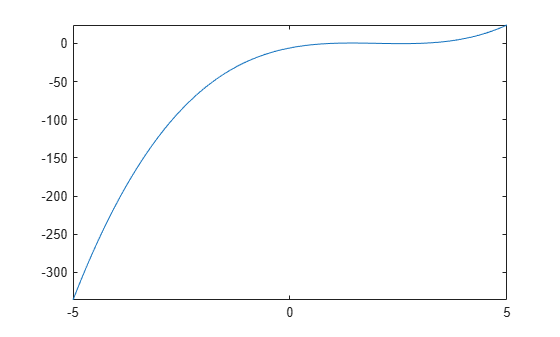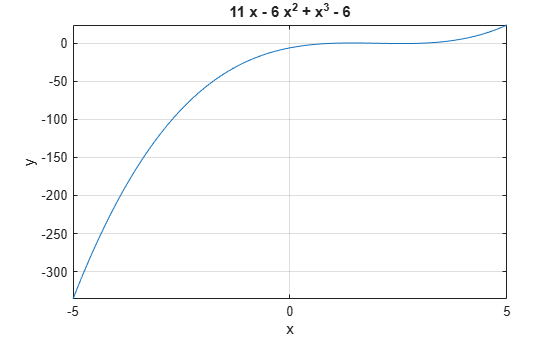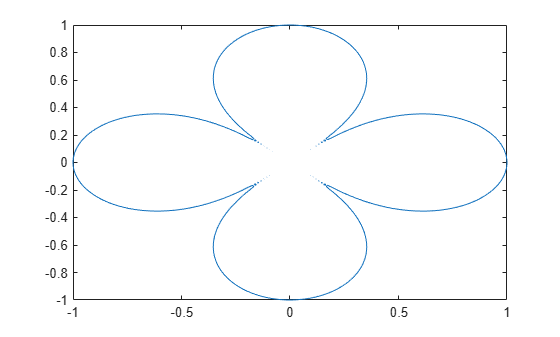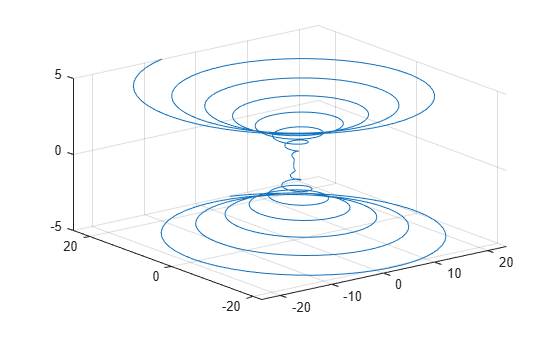## Perform Symbolic Computations

### Differentiate Symbolic Expressions

With the Symbolic Math Toolbox™ software, you can find

• Derivatives of single-variable expressions

• Partial derivatives

• Second and higher order derivatives

• Mixed derivatives

For in-depth information on taking symbolic derivatives see Differentiation.

#### Expressions with One Variable

To differentiate a symbolic expression, use the `diff` command. The following example illustrates how to take a first derivative of a symbolic expression:

```syms x f = sin(x)^2; diff(f)```
```ans = 2*cos(x)*sin(x)```

#### Partial Derivatives

For multivariable expressions, you can specify the differentiation variable. If you do not specify any variable, MATLAB® chooses a default variable by its proximity to the letter `x`:

```syms x y f = sin(x)^2 + cos(y)^2; diff(f)```
```ans = 2*cos(x)*sin(x)```

For the complete set of rules MATLAB applies for choosing a default variable, see Find a Default Symbolic Variable.

To differentiate the symbolic expression `f` with respect to a variable `y`, enter:

```syms x y f = sin(x)^2 + cos(y)^2; diff(f, y)```
```ans = -2*cos(y)*sin(y)```

#### Second Partial and Mixed Derivatives

To take a second derivative of the symbolic expression `f` with respect to a variable `y`, enter:

```syms x y f = sin(x)^2 + cos(y)^2; diff(f, y, 2)```
```ans = 2*sin(y)^2 - 2*cos(y)^2```

You get the same result by taking derivative twice: ```diff(diff(f, y))```. To take mixed derivatives, use two differentiation commands. For example:

```syms x y f = sin(x)^2 + cos(y)^2; diff(diff(f, y), x)```
```ans = 0```

### Integrate Symbolic Expressions

You can perform symbolic integration including:

• Indefinite and definite integration

• Integration of multivariable expressions

For in-depth information on the `int` command including integration with real and complex parameters, see Integration.

#### Indefinite Integrals of One-Variable Expressions

Suppose you want to integrate a symbolic expression. The first step is to create the symbolic expression:

```syms x f = sin(x)^2;```

To find the indefinite integral, enter

`int(f)`
```ans = x/2 - sin(2*x)/4```

#### Indefinite Integrals of Multivariable Expressions

If the expression depends on multiple symbolic variables, you can designate a variable of integration. If you do not specify any variable, MATLAB chooses a default variable by the proximity to the letter `x`:

```syms x y n f = x^n + y^n; int(f)```
```ans = x*y^n + (x*x^n)/(n + 1)```

For the complete set of rules MATLAB applies for choosing a default variable, see Find a Default Symbolic Variable.

You also can integrate the expression `f = x^n + y^n` with respect to `y`

```syms x y n f = x^n + y^n; int(f, y)```
```ans = x^n*y + (y*y^n)/(n + 1)```

If the integration variable is `n`, enter

```syms x y n f = x^n + y^n; int(f, n)```
```ans = x^n/log(x) + y^n/log(y)```

#### Definite Integrals

To find a definite integral, pass the limits of integration as the final two arguments of the `int` function:

```syms x y n f = x^n + y^n; int(f, 1, 10)```
```ans = piecewise(n == -1, log(10) + 9/y, n ~= -1,... (10*10^n - 1)/(n + 1) + 9*y^n)```

#### If MATLAB Cannot Find a Closed Form of an Integral

If the `int` function cannot compute an integral, it returns an unresolved integral:

```syms x int(sin(sinh(x)))```
```ans = int(sin(sinh(x)), x)```

### Solve Equations

You can solve different types of symbolic equations including:

• Algebraic equations with one symbolic variable

• Algebraic equations with several symbolic variables

• Systems of algebraic equations

For in-depth information on solving symbolic equations including differential equations, see Equation Solving.

#### Solve Algebraic Equations with One Symbolic Variable

Use the double equal sign (==) to define an equation. Then you can `solve` the equation by calling the solve function. For example, solve this equation:

```syms x solve(x^3 - 6*x^2 == 6 - 11*x)```
```ans = 1 2 3```

If you do not specify the right side of the equation, `solve` assumes that it is zero:

```syms x solve(x^3 - 6*x^2 + 11*x - 6)```
```ans = 1 2 3```

#### Solve Algebraic Equations with Several Symbolic Variables

If an equation contains several symbolic variables, you can specify a variable for which this equation should be solved. For example, solve this multivariable equation with respect to `y`:

```syms x y solve(6*x^2 - 6*x^2*y + x*y^2 - x*y + y^3 - y^2 == 0, y)```
```ans = 1 2*x -3*x```

If you do not specify any variable, you get the solution of an equation for the alphabetically closest to `x` variable. For the complete set of rules MATLAB applies for choosing a default variable see Find a Default Symbolic Variable.

#### Solve Systems of Algebraic Equations

You also can solve systems of equations. For example:

```syms x y z [x, y, z] = solve(z == 4*x, x == y, z == x^2 + y^2)```
```x = 0 2 y = 0 2 z = 0 8```

### Simplify Symbolic Expressions

Symbolic Math Toolbox provides a set of simplification functions allowing you to manipulate the output of a symbolic expression. For example, the following polynomial of the golden ratio `phi`

```phi = (1 + sqrt(sym(5)))/2; f = phi^2 - phi - 1```

returns

```f = (5^(1/2)/2 + 1/2)^2 - 5^(1/2)/2 - 3/2```

You can simplify this answer by entering

`simplify(f)`

and get a very short answer:

```ans = 0```

Symbolic simplification is not always so straightforward. There is no universal simplification function, because the meaning of a simplest representation of a symbolic expression cannot be defined clearly. Different problems require different forms of the same mathematical expression. Knowing what form is more effective for solving your particular problem, you can choose the appropriate simplification function.

For example, to show the order of a polynomial or symbolically differentiate or integrate a polynomial, use the standard polynomial form with all the parentheses multiplied out and all the similar terms summed up. To rewrite a polynomial in the standard form, use the `expand` function:

```syms x f = (x ^2- 1)*(x^4 + x^3 + x^2 + x + 1)*(x^4 - x^3 + x^2 - x + 1); expand(f)```
```ans = x^10 - 1```

The `factor` simplification function shows the polynomial roots. If a polynomial cannot be factored over the rational numbers, the output of the `factor` function is the standard polynomial form. For example, to factor the third-order polynomial, enter:

```syms x g = x^3 + 6*x^2 + 11*x + 6; factor(g)```
```ans = [ x + 3, x + 2, x + 1]```

The nested (Horner) representation of a polynomial is the most efficient for numerical evaluations:

```syms x h = x^5 + x^4 + x^3 + x^2 + x; horner(h)```
```ans = x*(x*(x*(x*(x + 1) + 1) + 1) + 1)```

For a list of Symbolic Math Toolbox simplification functions, see Choose Function to Rearrange Expression.

### Substitutions in Symbolic Expressions

#### Substitute Symbolic Variables with Numbers

You can substitute a symbolic variable with a numeric value by using the `subs` function. For example, evaluate the symbolic expression `f` at the point `x` = 1/3:

```syms x f = 2*x^2 - 3*x + 1; subs(f, 1/3)```
```ans = 2/9```

The `subs` function does not change the original expression `f`:

`f`
```f = 2*x^2 - 3*x + 1```

#### Substitute in Multivariate Expressions

When your expression contains more than one variable, you can specify the variable for which you want to make the substitution. For example, to substitute the value `x` = 3 in the symbolic expression

```syms x y f = x^2*y + 5*x*sqrt(y);```

enter the command

`subs(f, x, 3)`
```ans = 9*y + 15*y^(1/2)```

#### Substitute One Symbolic Variable for Another

You also can substitute one symbolic variable for another symbolic variable. For example to replace the variable `y` with the variable `x`, enter

`subs(f, y, x)`
```ans = x^3 + 5*x^(3/2)```

#### Substitute a Matrix into a Polynomial

You can also substitute a matrix into a symbolic polynomial with numeric coefficients. There are two ways to substitute a matrix into a polynomial: element by element and according to matrix multiplication rules.

Element-by-Element Substitution.  To substitute a matrix at each element, use the `subs` command:

```syms x f = x^3 - 15*x^2 - 24*x + 350; A = [1 2 3; 4 5 6]; subs(f,A)```
```ans = [ 312, 250, 170] [ 78, -20, -118]```

You can do element-by-element substitution for rectangular or square matrices.

Substitution in a Matrix Sense.  If you want to substitute a matrix into a polynomial using standard matrix multiplication rules, a matrix must be square. For example, you can substitute the magic square `A` into a polynomial `f`:

1. Create the polynomial:

```syms x f = x^3 - 15*x^2 - 24*x + 350;```
2. Create the magic square matrix:

`A = magic(3)`
```A = 8 1 6 3 5 7 4 9 2```
3. Get a row vector containing the numeric coefficients of the polynomial `f`:

`b = sym2poly(f)`
```b = 1 -15 -24 350```
4. Substitute the magic square matrix `A` into the polynomial `f`. Matrix `A` replaces all occurrences of `x` in the polynomial. The constant times the identity matrix `eye(3)` replaces the constant term of `f`:

`A^3 - 15*A^2 - 24*A + 350*eye(3)`
```ans = -10 0 0 0 -10 0 0 0 -10```

The `polyvalm` command provides an easy way to obtain the same result:

`polyvalm(b,A)`
```ans = -10 0 0 0 -10 0 0 0 -10```

#### Substitute the Elements of a Symbolic Matrix

To substitute a set of elements in a symbolic matrix, also use the `subs` command. Suppose you want to replace some of the elements of a symbolic circulant matrix A

```syms a b c A = [a b c; c a b; b c a]```
```A = [ a, b, c] [ c, a, b] [ b, c, a]```

To replace the (2, 1) element of `A` with `beta` and the variable `b` throughout the matrix with variable `alpha`, enter

```alpha = sym('alpha'); beta = sym('beta'); A(2,1) = beta; A = subs(A,b,alpha)```

The result is the matrix:

```A = [ a, alpha, c] [ beta, a, alpha] [ alpha, c, a]```

### Plot Symbolic Functions

Symbolic Math Toolbox provides the plotting functions:

#### Explicit Function Plot

Create a 2-D line plot by using `fplot`. Plot the expression ${x}^{3}-6{x}^{2}+11x-6$.

```syms x f = x^3 - 6*x^2 + 11*x - 6; fplot(f)```Add labels for the x- and y-axes. Generate the title by using `texlabel(f)`. Show the grid by using `grid on`. For details, see Add Title and Axis Labels to Chart.

```xlabel('x') ylabel('y') title(texlabel(f)) grid on```#### Implicit Function Plot

Plot equations and implicit functions using `fimplicit`.

Plot the equation $\left({x}^{2}+{y}^{2}{\right)}^{4}=\left({x}^{2}-{y}^{2}{\right)}^{2}$ over $-1.

```syms x y eqn = (x^2 + y^2)^4 == (x^2 - y^2)^2; fimplicit(eqn, [-1 1])```#### 3-D Plot

Plot 3-D parametric lines by using `fplot3`.

Plot the parametric line

`$\begin{array}{c}x={t}^{2}\mathrm{sin}\left(10t\right)\\ y={t}^{2}\mathrm{cos}\left(10t\right)\\ z=t.\end{array}$`

```syms t fplot3(t^2*sin(10*t), t^2*cos(10*t), t)```#### Create Surface Plot

Create a 3-D surface by using `fsurf`.

Plot the paraboloid $z={x}^{2}+{y}^{2}$.

```syms x y fsurf(x^2 + y^2)```## Support

#### Mathematical Modeling with Symbolic Math Toolbox

Get examples and videos